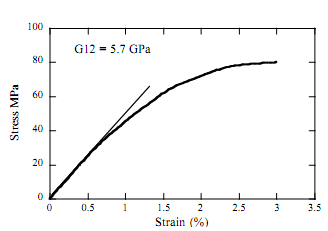## In-plane shear tests for composite materials , Other Engineering

Assignment Help:

The ideal test should provide a region of pure, uniform shear stress within the test sample.
The most simple test is the tension test on the (+/-45°)ns sample, where n is greater than one.

The specimen width is usually between 13 and 25 mm and strain gauges are bonded to the sample at 0° and 90°.
It can be shown that the shear stress in the sample, τ12,

Where σx is the stress in the longitudinal direction (=P/A).

The shear modulus, G12, is determined by plotting the shear stress against the shear strain and measuring the slope of the resulting curve.#### Numericalle.., The Stefan-Boltzmann law can be employed to estimate the rat...

The Stefan-Boltzmann law can be employed to estimate the rate of radiation of energy H from a surface of copper sphere with radius = 0.15 ±0.01 m, as in H=AesT^4 where H is in watt

#### Heat transfer, Q. 1 A 50 cm diameter pipeline in the Arctic Carries hot oi...

Q. 1 A 50 cm diameter pipeline in the Arctic Carries hot oil at 30 0C and exposed to a coefficient surrounding temperature of -20 0C. Special powder insulation 5 cm thick surround

#### Operational amplifier, ideal and practical amplifier

ideal and practical amplifier

#### Function, what is function independence.on software engineering

what is function independence.on software engineering

#### Siliconizing, 1.defination 2.explain the mechanisms of each process. 3. exp...

1.defination 2.explain the mechanisms of each process. 3. explain in detail how many types of each process 4. advantages 5. disadvantages

#### Material used for patterns, Material Used for Patterns : The common materi...

Material Used for Patterns : The common materials of which the patterns are made of the following :             1. Wood: The common woods are :             (i)   Pine

#### Value analysis, what is value analysis

what is value analysis

#### Production system, Garden Variety Flower Shop uses 750 clay pots a month. ...

Garden Variety Flower Shop uses 750 clay pots a month. The pots are purchased at \$2 each. Annual carrying costs are estimated to be 25 percent of cost, and ordering costs are \$30

#### Electrochemistry, can two metals such as iron and nickel be separated compl...

can two metals such as iron and nickel be separated completely by electrogravimetry?

#### X rays., how x rays are produced?

how x rays are produced?